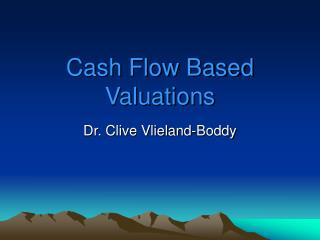DownloadDownload PresentationCash Flow Based Valuations

# Cash Flow Based Valuations

Télécharger la présentation## Cash Flow Based Valuations

- - - - - - - - - - - - - - - - - - - - - - - - - - - E N D - - - - - - - - - - - - - - - - - - - - - - - - - - -
##### Presentation Transcript

1. Cash Flow Based Valuations Dr. Clive Vlieland-Boddy

2. Discounted Cash Flows • Project the cash flow stream • Chose a discount rate • Determine a terminal value • Calculate the present value

3. Discounted cash flow analysis • Cash receipts are estimated for each of several future periods. • These receipts are converted to value by the application of a discount rate using present value techniques. • The timing of cash inflows and cash outlays are important as well as the amount of the receipts. • The discount rate must be appropriate for the definition of cash flow used. where P0 = present value or market price r = investors’ required rate of return C1, C2, C3= expected income flows in periods 1, 2, 3

4. Example: Using the Discounted Future Income Method to Estimate the Value of a Business *Terminal Value Estimate

5. Principal difficulties with the discounted cash flow technique • Long-term cash flow projections are subject to substantial error. • The choice of the discounting horizon, the point of competitive equilibrium, is also uncertain. • The terminal value, which often accounts for 70% of the final valuation, is subject to the highest error

6. Weighted Average Cost of Capital (WACC)

7. Matching the Discount Rate to the Income Stream For a growing business, net cash flow is typically projected as follows: Net income (after tax) + Non-cash charges (e.g. Depreciation and amortization) • Cash needed for asset replacement - Increase in working capital needed as the business grows + Increase in debt to finance business growth

8. Matching the Discount Rate to the Income Stream (cont’d) • Cash needed for asset replacement will generally exceed depreciation and amortization. • Therefore, when net income is used as the income stream, an upward adjustment should be made to the discount or capitalization rate. • This adjustment should be proportionate to the ratio of net income to net cash flow. • For example, assume net income is \$60,000 and net cash flow is \$50,000. Also assume the net cash flow discount rate is 20%. The net income discount rate would be 24% as follows: \$60,000/\$50,000 = 1.2 20% x 1.2 = 24% • Other valuers feel the difference between net cash flow and net income, in the long run, is usually small and can generally be ignored or included in the company-specific risk factor.

9. Discounted Cash-Flow Approach • Estimated future cash flows are discounted back to present value based on the investor’s required rate of return • Discounted dividend valuation • Discounted operating cash-flow models

10. Discounted Dividend Valuation • Most straightforward approach • Explicit cash flows received by equity investors • Dividends • Terminal value when shares are sold • Firm is expected to have an infinite life

11. Discounted Dividend ValuationRequired rate of return (r) • For nonpublic companies, use a buildup model and historical sources for data • Begin with risk-free rate • + Equity risk premium • + Small company premium • + Company specific risk premium • = Required rate of return

12. Discounted Dividend ValuationGrowth rate (g) • Top-Down analysis • Begin with growth of economy • Adjust for industry, sector and company factors • Sustainable growth = ROE(1-Payout rate) • ROE = Earnings/Average equity • Payout rate: proportion of earnings used to pay dividends or repurchase shares

13. FCF

14. Which method is best? (1) Balance sheet method? (2) Income statement multiples method? (3) Discounted cash flow method?